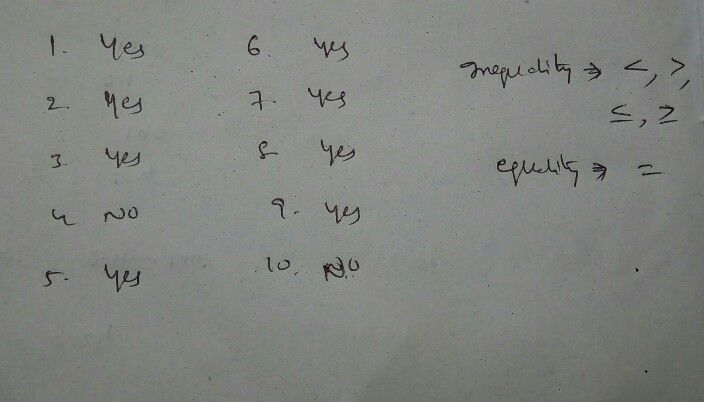Symbol
ProblemActivity $2s$ Quadratic Inequalities or Not? Tell whether the following mathematical sentence is quadratic inequality or not. Write YES if quadratic inequality and write NO if not. Write your answer on the blanks. $1.2x^{2+3x+2}<0$ $6.y$ $x^{2}-4x-32\leq 0$ $2.$ $x^{2}-3x-10>0$ $7,$ $2x^{2}-4x-48>0$ $3.\left(2x-5\right)\left(x+4\right)\geq 0$ 8. $2x+5x>0$ $4$ $x^{2}-4x-12=0$ $9.3$ $3x^{2+15x-30}\geq 0$ $5$ $5x-24<0$ $10$ $x2+3=0$
7th-9th grade
Other
Search count: 106
SolutionQanda teacher - premsagarStudent
if the answer is no can u please write the answer?